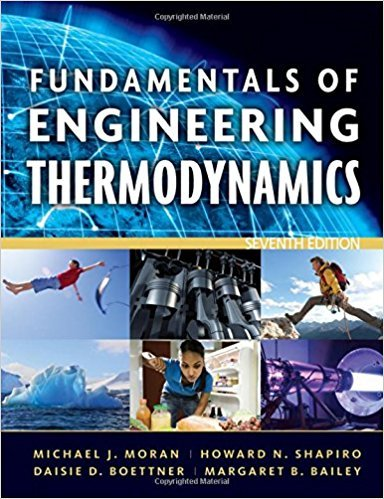×
Get Full Access to Fundamentals Of Engineering Thermodynamics - 7 Edition - Chapter 4 - Problem 13p
Get Full Access to Fundamentals Of Engineering Thermodynamics - 7 Edition - Chapter 4 - Problem 13p

×

# Applying Conservation of MassAs shown in Fig. P4.13, steamISBN: 9780470495902 50

## Solution for problem 13P Chapter 4

Fundamentals of Engineering Thermodynamics | 7th Edition

• Textbook Solutions
• 2901 Step-by-step solutions solved by professors and subject experts
• Get 24/7 help from StudySoup virtual teaching assistantsFundamentals of Engineering Thermodynamics | 7th Edition

4 5 1 239 Reviews
30
4
Problem 13P

Problem 13P

Applying Conservation of Mass

As shown in Fig. P4.13, steam at 80 bar, 440°C, enters a turbine operating at steady state with a volumetric flow rate of 236 m3/min. Twenty percent of the entering mass flow exits through a diameter of 0.25 m at 60 bar, 400°C. The rest exits through a diameter of 1.5 m with a pressure of 0.7 bar and a quality of 90%. Determine the velocity at each exit duct, in m/s.Fig. P4.13

Step-by-Step Solution:

Solution 13P

Step 1 of 8:

In this question, we need to find the velocity of steam out of each exit duct of the turbine

Data given

Intel pressureInitial temperatureVelocity of steam into the turbineMass of  steam from exitTemperature at outletPressure at outletDiameter of outlet ductQualityPressure at two exit ductDiameter of second exit ductStep 2 of 8

Step 3 of 8

##### ISBN: 9780470495902

Since the solution to 13P from 4 chapter was answered, more than 1673 students have viewed the full step-by-step answer. This full solution covers the following key subjects: bar, fig, flow, mass, diameter. This expansive textbook survival guide covers 14 chapters, and 1501 solutions. Fundamentals of Engineering Thermodynamics was written by and is associated to the ISBN: 9780470495902. The answer to “Applying Conservation of MassAs shown in Fig. P4.13, steam at 80 bar, 440°C, enters a turbine operating at steady state with a volumetric flow rate of 236 m3/min. Twenty percent of the entering mass flow exits through a diameter of 0.25 m at 60 bar, 400°C. The rest exits through a diameter of 1.5 m with a pressure of 0.7 bar and a quality of 90%. Determine the velocity at each exit duct, in m/s. Fig. P4.13” is broken down into a number of easy to follow steps, and 77 words. The full step-by-step solution to problem: 13P from chapter: 4 was answered by , our top Engineering and Tech solution expert on 07/20/17, 09:01AM. This textbook survival guide was created for the textbook: Fundamentals of Engineering Thermodynamics, edition: 7.

Unlock Textbook Solution